Constructing a triangle when 3 sides known (SSS)

Practical Geometry
Concept wise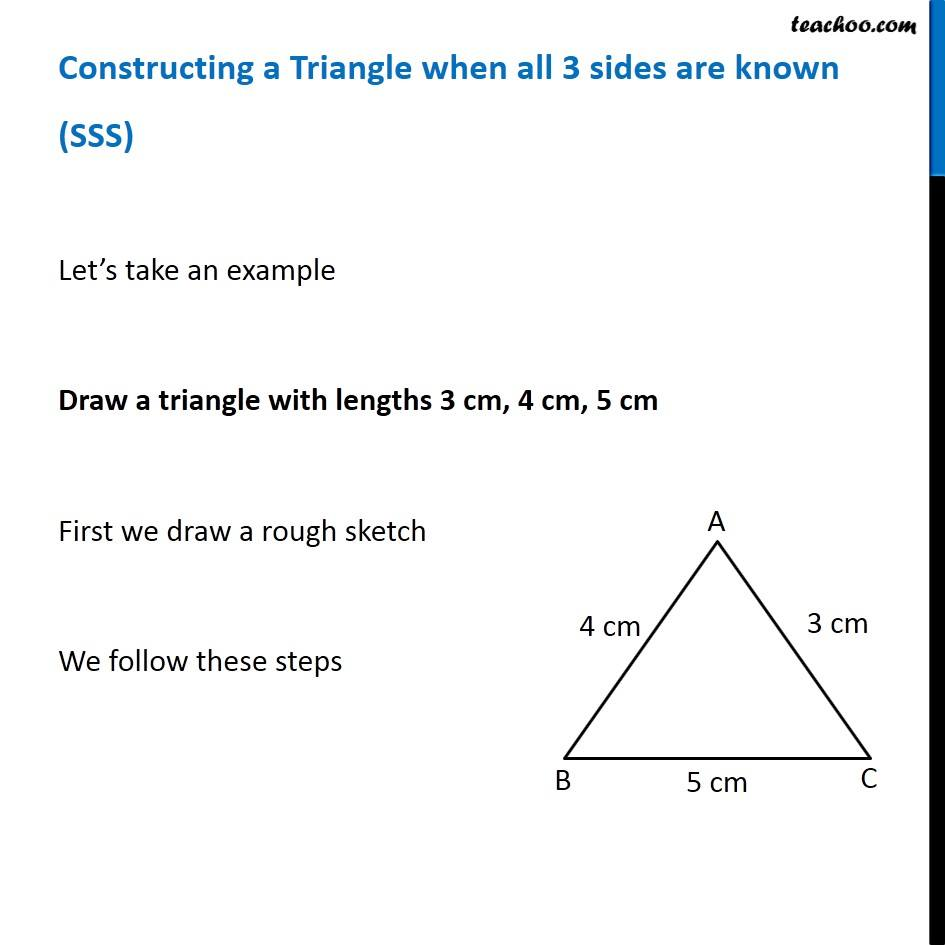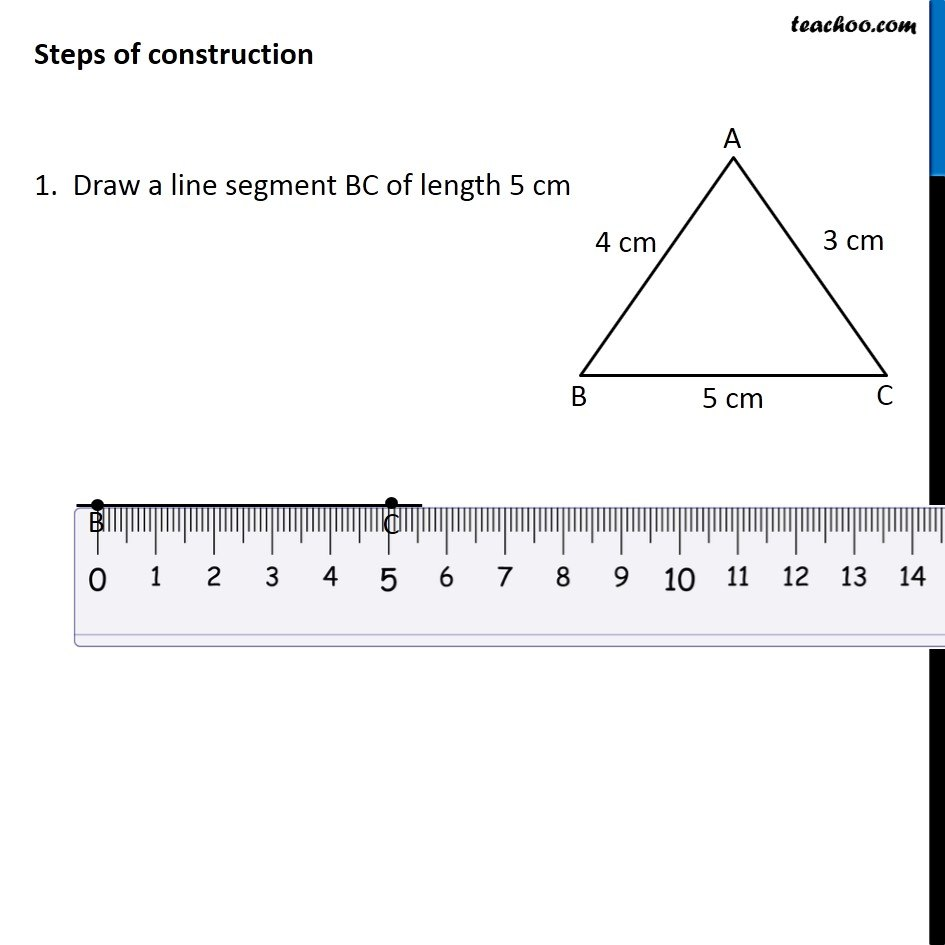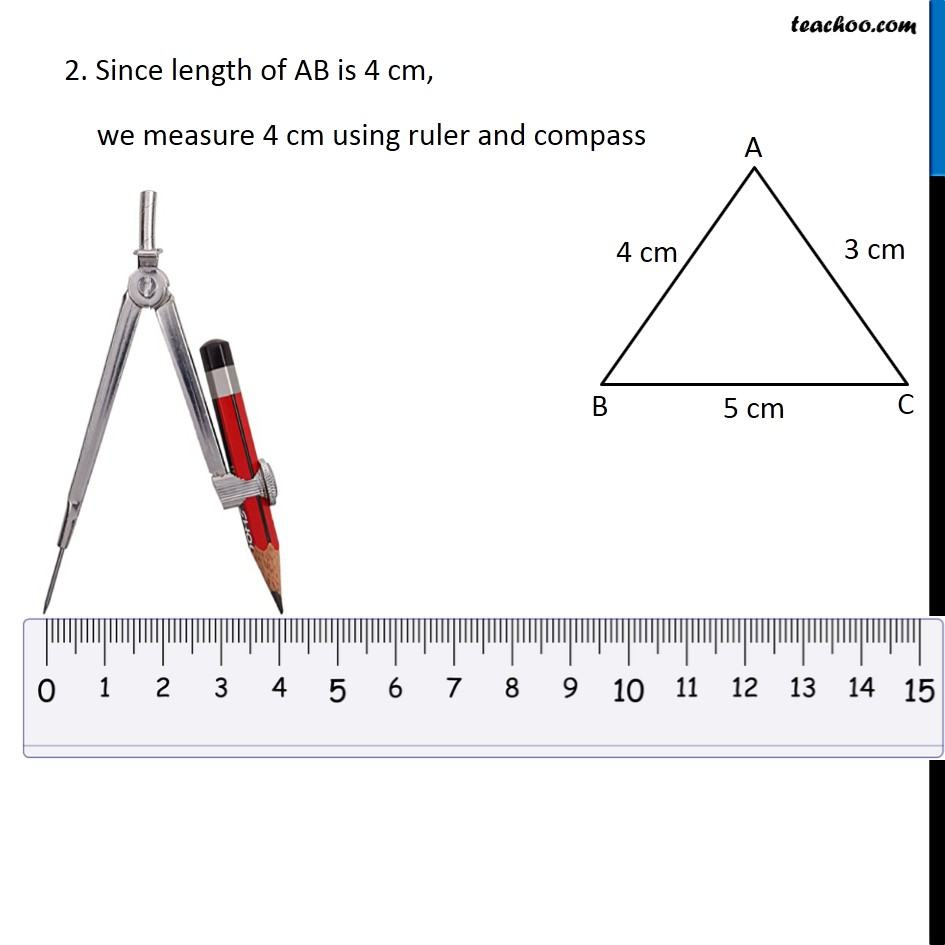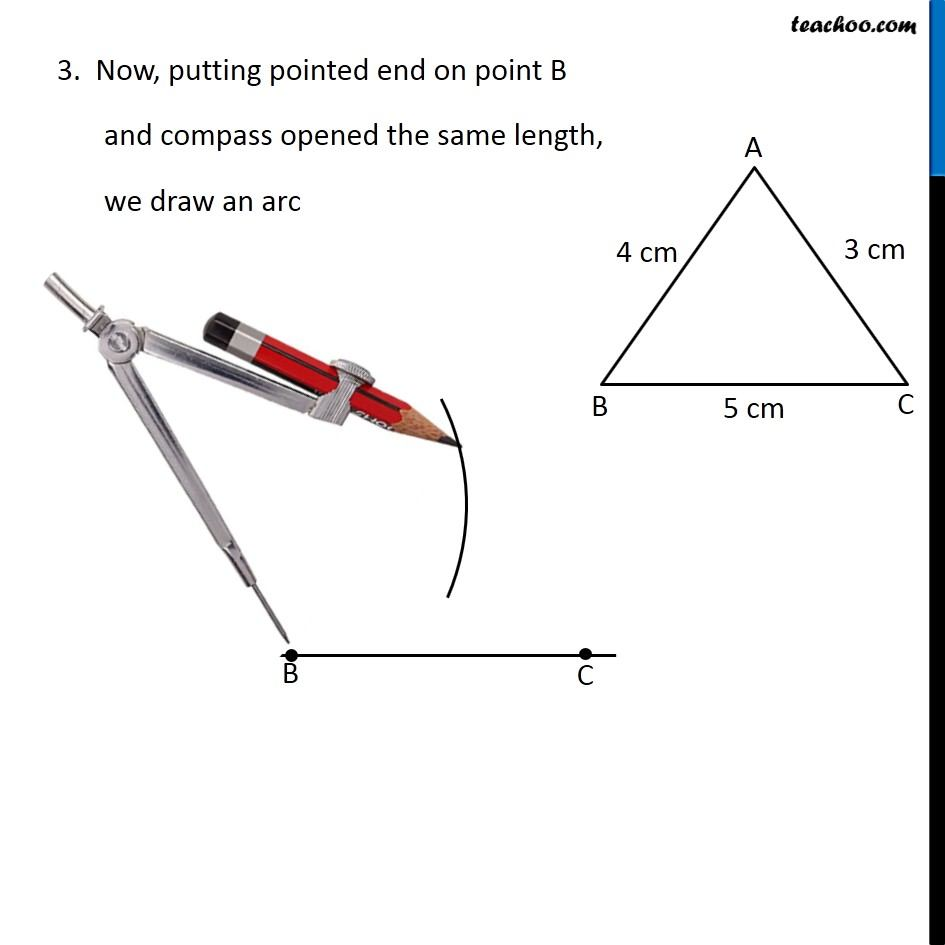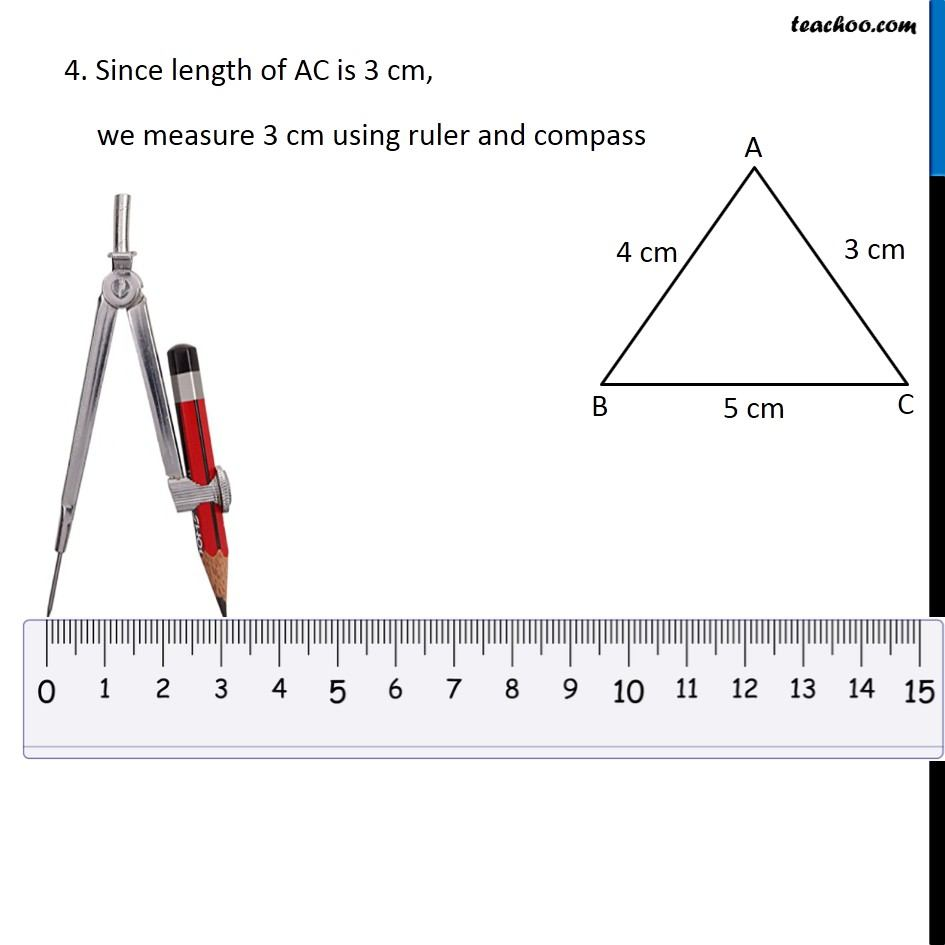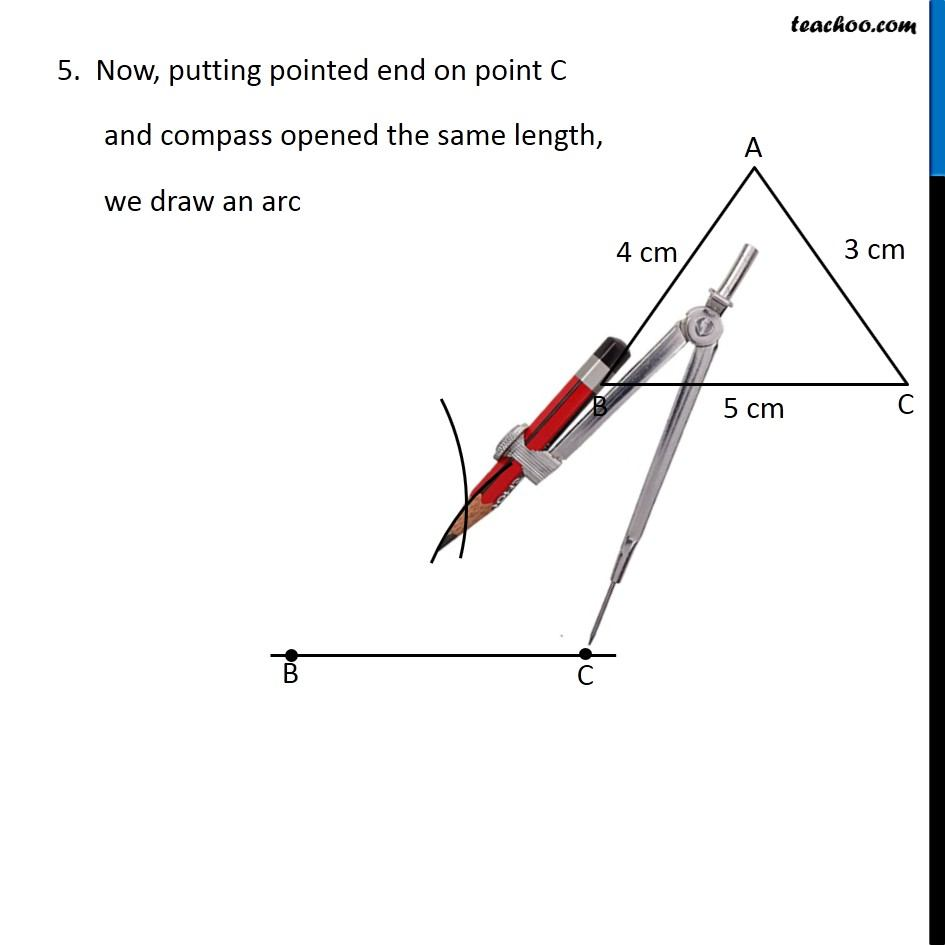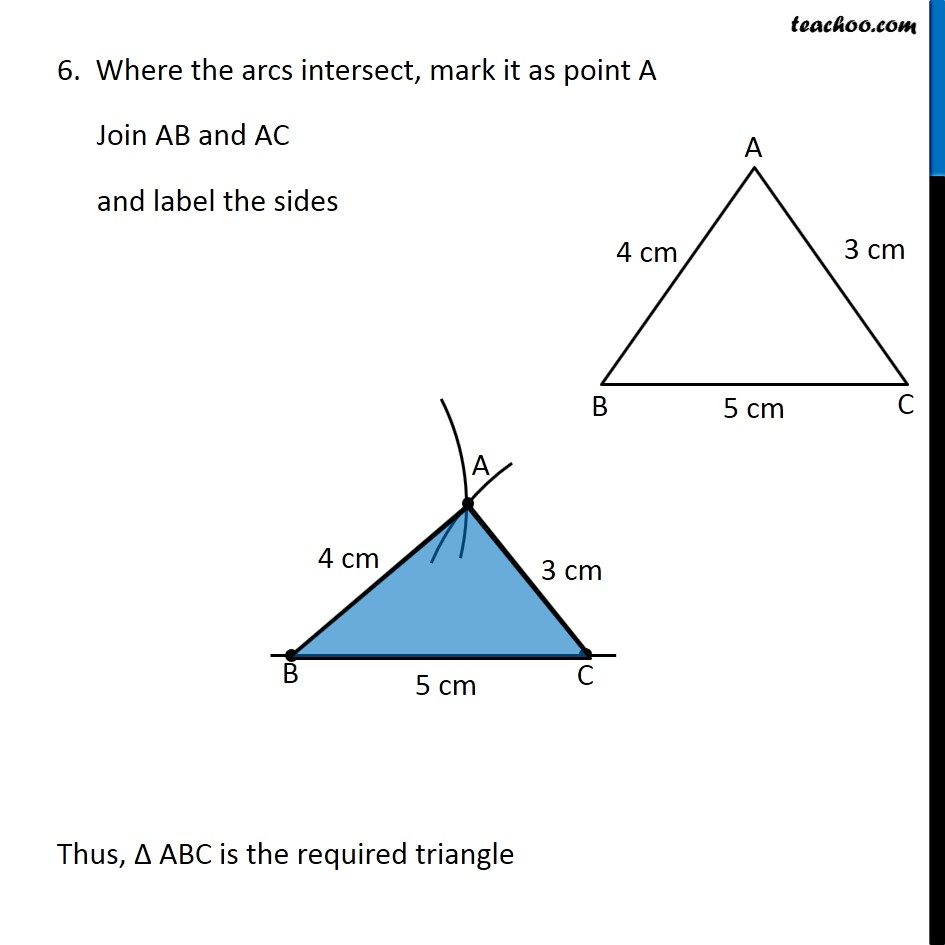Learn in your speed, with individual attention - Teachoo Maths 1-on-1 Class

### Transcript

Constructing a Triangle when all 3 sides are known (SSS) Let’s take an example Draw a triangle with lengths 3 cm, 4 cm, 5 cm First we draw a rough sketch We follow these steps Steps of construction 1. Draw a line segment BC of length 5 cm 2. Since length of AB is 4 cm, we measure 4 cm using ruler and compass 3. Now, putting pointed end on point B and compass opened the same length, we draw an arc 4. Since length of AC is 3 cm, we measure 3 cm using ruler and compass 5. Now, putting pointed end on point C and compass opened the same length, we draw an arc 6. Where the arcs intersect, mark it as point A Join AB and AC and label the sides Thus, Δ ABC is the required triangle